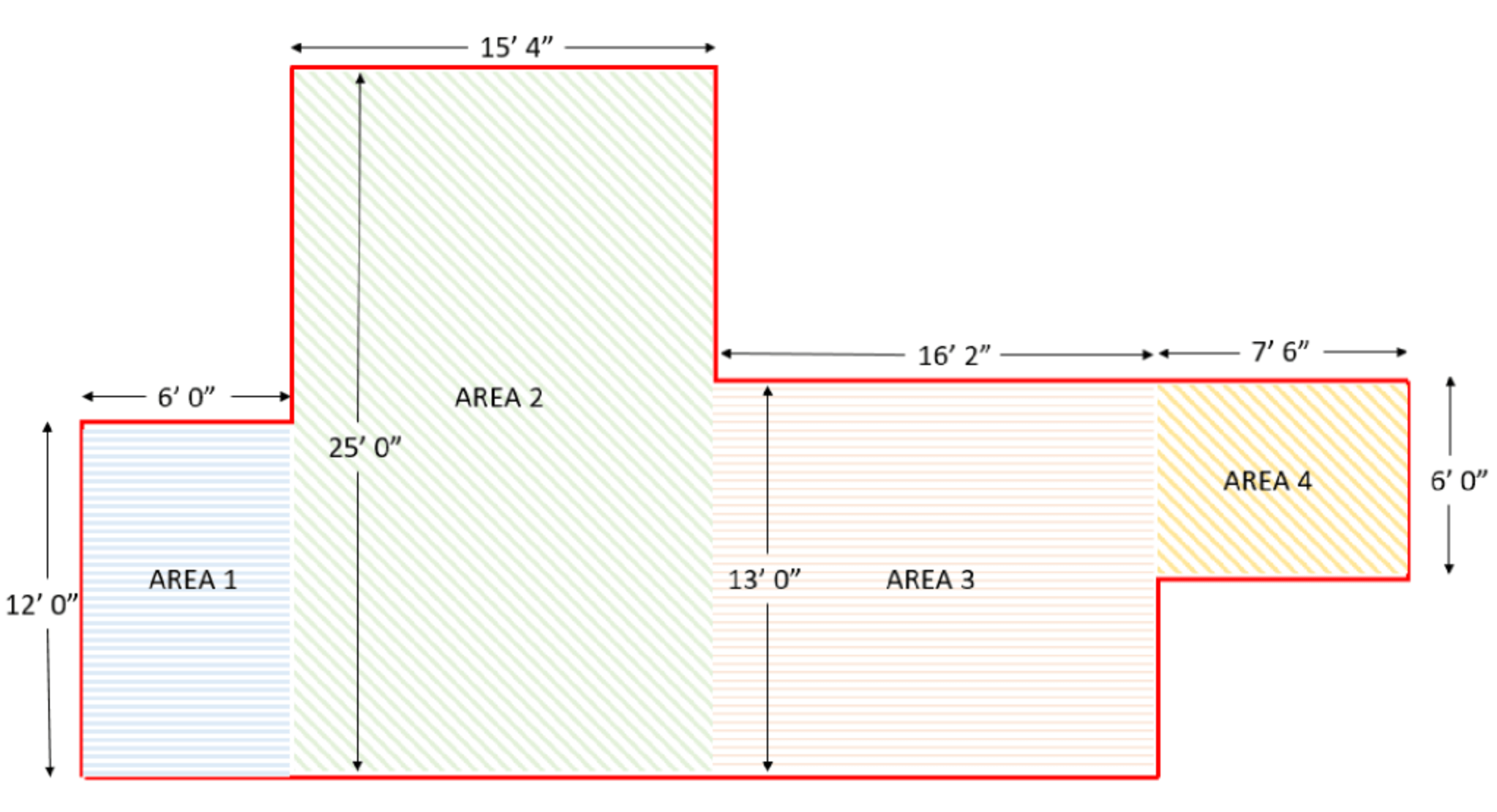# Holloway Hickory

\$1.69 \$2.49

12mm

Per Box - 14.13 Sq Ft

see our prices
Calculate my Footage Calculate my Square Footage
To calculate feet of trim, (e.g. base, quarter round) simply measure each wall in the room and add up the linear feet. For ease of installation use whole pieces where ever possible. Allow extra for cutting each corner and splicing pieces together.To determine the square footage of a square or rectangular room, measure the length and width of the room in feet or inches and enter the values in Area 1 of the calculator below.
x
You'll need Sq Ft
1 Boxes • covers 23 Sq Ft
How To Measure Multiple Spaces +
To determine the square footage of a square or rectangular room, measure the length and width of the room in feet or inches and enter the values in Area 1 of the calculator below. For irregularly shaped areas or multiple rooms, it is helpful to draw a diagram and divide the room up into sections. Treat each section as a separate area, as in this illustration. Input the measurements of each area below to see the total SQFT needed.